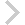FICO Xpress Optimization Examples Repository
 FICO Optimization Community FICO Xpress Optimization HomePplan: A project planning problem

Description
Over the next 6 months we have three projects which can be done. Each of these projects has a profile of manpower requirements over its lifetime, and a benefit which accrues each month when the project has been completed. Our problem is to determine when each project is to start, subject to the constraint that in no month can we try to use more manpower than is available.

Further explanation of this example: 'Xpress Python Reference Manual'

Source Files
By clicking on a file name, a preview is opened at the bottom of this page.

pplan.py

'''*******************************************************
* Python Example Problems                             *
*                                                     *
* file pplan.py                                       *
* Example for the use of the Python language          *
* (Manpower planning problem)                         *
*                                                     *
* (c) 2019-2023 Fair Isaac Corporation                *
*******************************************************'''

from __future__ import print_function
import xpress as xp

DUR = [3, 3, 4]
RESMAX = [5, 6, 7, 7, 6, 6]
BEN = [10.2, 12.3, 11.2]
RESUSE = [[3, 4, 2, 0, 0, 0], [4, 1, 5, 0, 0, 0], [3, 2, 1, 2, 0, 0]]

RProj = range(3)
NTime = 6
RTime = range(NTime)

x = xp.vars(RProj, RTime, vartype=xp.binary)
start = [xp.var(ub=NTime - DUR[p] + 1) for p in RProj]

# Objective, to be maximized: Benefit.  If project p starts in month
# t, it finishes in month t+DUR(p)-1 and contributes a benefit of
# BEN(p) for the remaining NTime-(t+DUR(p)-1) months.
MaxBen = xp.Sum(BEN[p]*(NTime-t-DUR[p]+1) * x[p, t]
for p in RProj for t in range(NTime - DUR[p]))

prob = xp.problem()

# Resource availability
# A project starting in month s is in its t-s+1'st month in month t:
for p in RProj for s in range(t+1))
<= RESMAX[t] for t in RTime)

# Logical Constraints: Each project starts once and only once:
prob.addConstraint(xp.Sum(x[p, t] for t in RTime) == 1 for p in RProj)

# Connect variables x(p,t) and start(p)
prob.addConstraint(xp.Sum(t*x[p, t] for t in RTime) == start[p] for p in RProj)

# Finish everything by the end of the planning period
prob.addConstraint(start[p] <= NTime - DUR[p] + 1 for p in RProj)

prob.setObjective(MaxBen, sense=xp.maximize)

prob.optimize()

print(" Objective: ", prob.getObjVal())

print('    ', end='')
for t in RTime:
print("{:5d}".format(t), end='')

print('')
for p in RProj:
print('{:3d}:'.format(p), end='')
for t in RTime:
sol = prob.getSolution(start[p])
if t < sol:
char = ' '
elif t < sol + DUR[p]:
char = '*'
else:
char = 'B'
print("    {}".format(char), end='')
print('')